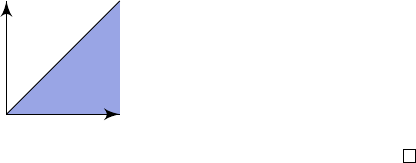5Transform theory

IB Complex Methods5.6 The convolution theorem for Laplace transforms
Finally, we recall from IB Methods that the Fourier transforms turn convolutions
into products, and vice versa. We will now prove an analogous result for Laplace
transforms.
Definition
(Convolution)
.
The convolution of two functions
f
and
g
is defined
as
(f g)(t) =
Z
−∞
f(t t
0
)g(t
0
) dt
0
.
When f and g vanish for negative t, this simplifies to
(f g)(t) =
Z
t
0
f(t t
0
)g(t) dt.
Theorem
(Convolution theorem)
.
The Laplace transform of a convolution is
given by
L(f g)(p) =
ˆ
f(p)ˆg(p).
Proof.
L(f g)(p) =
Z
0
Z
t
0
f(t t
0
)g(t
0
) dt
0
e
pt
dt
We change the order of integration in the (
t, t
0
) plane, and adjust the limits
accordingly (see picture below)
=
Z
0
Z
t
0
f(t t
0
)g(t
0
)e
pt
dt
dt
0
We substitute u = t t
0
to get
=
Z
0
Z
0
f(u)g(t
0
)e
pu
e
pt
0
du
dt
0
=
Z
0
Z
0
f(u)e
pu
du
g(t
0
)e
pt
0
dt
0
=
ˆ
f(p)ˆg(p).
Note the limits of integration are correct since they both represent the region
below
t
t
0